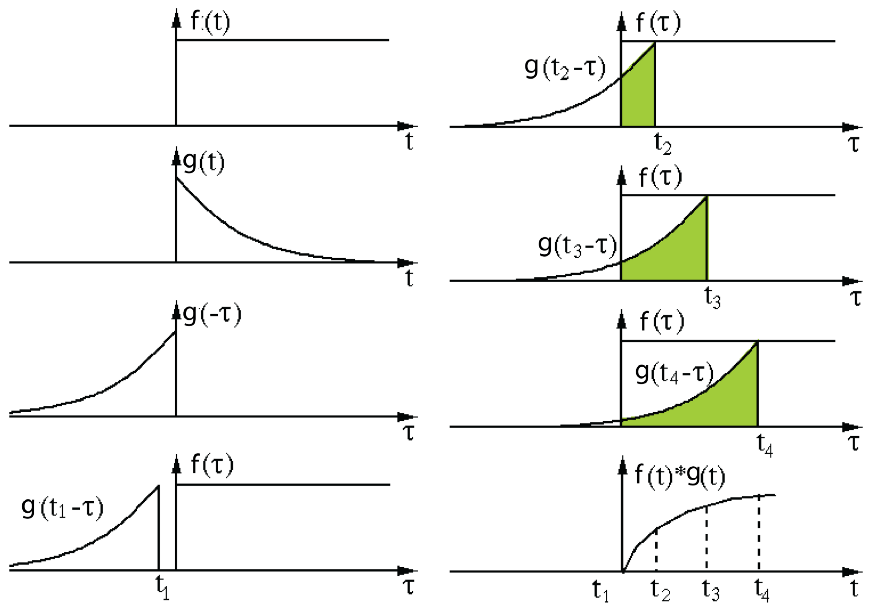# Convolution for Computer Science People

Knowing the concept of Convolution became crucial for computer science people, especially data scientists when convolution neural networks significantly improved vision tasks.

The convolution operator says to keep one function fixed, then move the other one over the fixed one and multiply them. This action results in a new function and calculates an integral for it or finds their sum if we are in the discrete realm.

The following figure shows how the convolution operation will flip one function and shift it over the fixed function.g(t) function is reversed concerning the y axis (because it has minus inside its parenthesis) [reference]

When the number of independent variables in functions increases, like for two variable functions like images:

So, in these cases, the flipping happens regarding both variables.

The following figures show how Convolution shifts a flipped function over the other.

As the functions are structurally symmetric in these examples, the flipping is not apparent.

## Important characteristics of Convolution

P1: Communitive property

P2: Associative property

P3: Identity property:

P4: Linear superposition and scaling properties

P5: Differentiation

Note: Usually for solving convolutions get help from the Laplace theorem by mapping from time to the frequency realm.

There is another function (cross-correlation) very similar to Convolution. Its difference is that it is not using the flipped version of the kernel. Instead, it moves the kernel over an image to find the similarity between the kernel (it can be a part of that image) and the fixed image (background image).

But, Convolution is used to change the pictures, usually like finding edges. If the kernel is symmetric, there is no difference between Convolution and correlation. Also, the cross correlation is defined and can be used as Convolution just by flipping the kernel.

In the following snippet, I used filter2D from the python OpenCV package that does the correlation, then I flipped the kernel and did Convolution.

Look at the results and know the difference:

Note that for flipping the kernel, we could easily use an OpenCV method called cv2.flip(src_img).

--

--